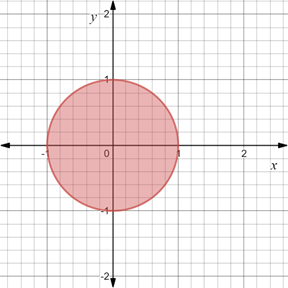# To graph : The region provided by the set { ( x , y ) | x 2 + y 2 ≤ 1 } .### Precalculus: Mathematics for Calcu...

6th Edition
Stewart + 5 others
Publisher: Cengage Learning
ISBN: 9780840068071### Precalculus: Mathematics for Calcu...

6th Edition
Stewart + 5 others
Publisher: Cengage Learning
ISBN: 9780840068071

#### Solutions

Chapter 1.8, Problem 109E
To determine

## To graph: The region provided by the set {(x,y)|x2+y2≤1} .

Expert Solution

### Explanation of Solution

Given information:

The set, {(x,y)|x2+y21} .

Graph:

The graph of the region provided by the set {(x,y)|x2+y21} is sketched in the coordinate plane.

Consider the equation x2+y2=1 .

Rewrite the equation as,

(x0)2+(y0)2=12

Recall that the standard form of the equation of the circle is (xh)2+(yk)2=r2 , where (h,k) denote the center of the circle and r denote the radius.

Compare, (xh)2+(yk)2=r2 and (x0)2+(y0)2=12 .

Here, h=0,k=0 and r=1 .

Therefore, center of circle is (0,0) and radius is 1 .

Now, on the Cartesian plane construct a circle with center at origin and radius 1 unit.

Since, the inequality is {(x,y)|x2+y21}

Now, shade the common region that lie interior to the circle..

The region obtained is provided below,Interpretation:

The region provided by the set {(x,y)|x2+y21} is shaded region. The region shaded forms a circle with center at origin and radius 1 unit. The shaded region is such that for each pair of point the condition x2+y21 should hold true. The region formed above is that radius of circle is less than or equal to 1.

### Have a homework question?

Subscribe to bartleby learn! Ask subject matter experts 30 homework questions each month. Plus, you’ll have access to millions of step-by-step textbook answers!